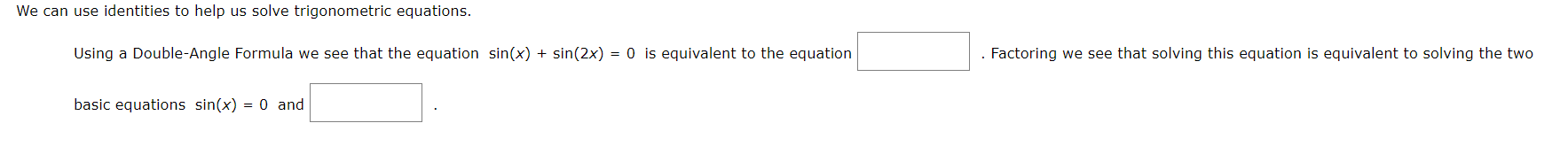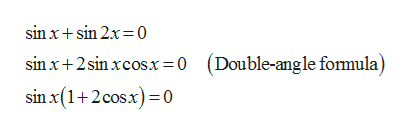# We can use identities to help us solve trigonometric equations.Using a Double-Angle Formula we see that the equation sin(x) sin(2x) = 0 is equivalent to the equation. Factoring we see that solving this equation is equivalent to solving the twobasic equations sin(x) = 0 and

Question
26 viewshelp_outlineImage TranscriptioncloseWe can use identities to help us solve trigonometric equations. Using a Double-Angle Formula we see that the equation sin(x) sin(2x) = 0 is equivalent to the equation . Factoring we see that solving this equation is equivalent to solving the two basic equations sin(x) = 0 and fullscreen
check_circle

Step 1

The given trigonometric equation is,

Step 2

The equivalent form of the...help_outlineImage Transcriptionclosesin xsin 2x 0 sin x+2 sin xcos.x = 0 (Double-angle formula) sin x(1+2cosx)=0 fullscreen

### Want to see the full answer?

See Solution

#### Want to see this answer and more?

Solutions are written by subject experts who are available 24/7. Questions are typically answered within 1 hour.*

See Solution
*Response times may vary by subject and question.
Tagged in

### Other